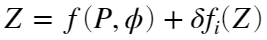## Conversion from water pressure to depth

バージョン 1.0.0 (1.99 KB) 作成者:
Leroy and Parthiot equation is used to the conversion of water pressure to depth. This equation is an alternative to the UNESCO equation.

ダウンロード: 44

ライセンスの表示

UNESCO equation to the conversion of water pressure to depth is widely used to oceanographers around the world. However, the Leroy and Parthiot equation () considers corrective terms, in function of various areas of the world; with this term, the water depth value reaches a better accuracy.
Inputs:
p = water pressure (in MPa, relative to atmospheric pressure).
lat = latitude of the point (in degrees).
D = number of area of applicability.
Output:
z = water depth (in m).
Example:
z = pre2depth(5,[30,45,60],0)
Reference:
Leroy, C. and Parthiot, F. 1998. Depth-pressure relationships in the oceans and seas. J. Acoust. Soc. Am., 103 (3), 1346-1352 p. DOI: 10.1121/1.421275

### 引用

Gabriel Ruiz-Martinez (2023). Conversion from water pressure to depth (https://www.mathworks.com/matlabcentral/fileexchange/105090-conversion-from-water-pressure-to-depth), MATLAB Central File Exchange. 取得済み .

すべてのリリースと互換性あり
##### プラットフォームの互換性
Windows macOS Linux

### Community Treasure Hunt

Find the treasures in MATLAB Central and discover how the community can help you!

Start Hunting!
バージョン 公開済み リリース ノート
1.0.0